Math Brain Teasers 6:: Solve the Picture Puzzle

in STEMGeekslast year (edited)

Hey All;

So are you guys ready for the Math Brain Teaser for today? We are now running into our 6th Math Brain Teaser competition. At the same time distributed close to 50 STEM tokens worth 11+ HIVE. Isn't that amazing?

The best part is we are seeing interest being developed in the StemGeeks community to solve these types of simple but fun-loving Math puzzles. The same can be noticed via the entries to the competition and the engagement level increasing trying to solve every puzzle that passes by.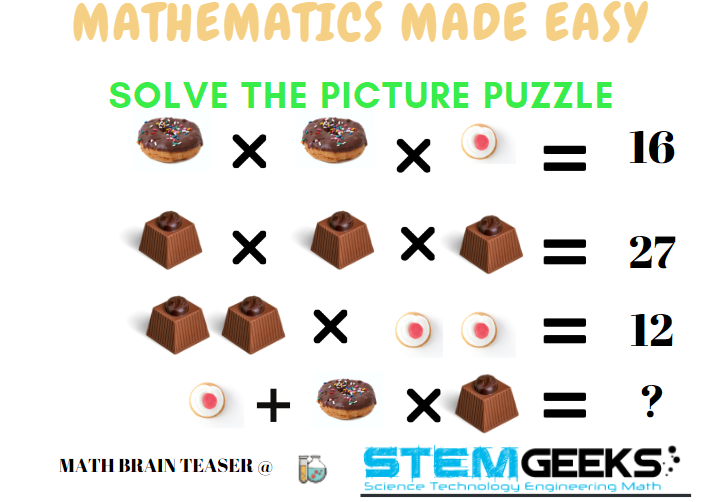Let's now get into our SIXTH:: Math Brain Teaser & this time we have donuts, chocolates, and cupcakes to eat and confuse you. I am sure you definitely like one of these items.. who doesn't like chocolates? Anyways carefully observe the picture above and try finding the answer to the fourth equation.

Hint:: Here is a simple hint to get you started. Give extra attention to the mathematics signs like multiplication & Addition. Also, there is a Mathematics Rule to be applied to get to the final answer. Make sure you are applying the RULE.

Math Brain Teasers 5:: Solve the Equation; What Do You Get? - Solved with Explanation

Let's have a look at the answers and the math/logic involved in finding:: Solve the Equation; What Do You Get? ? for our FIFTH Math Brain Teasers 5 Question.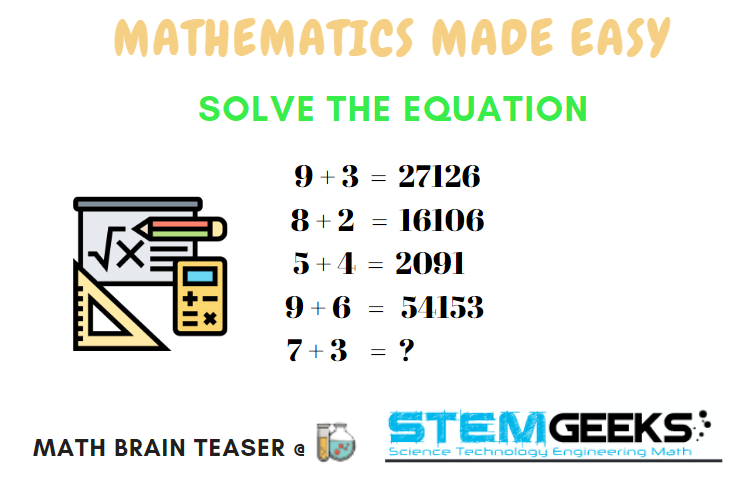Logic to Finding; Solve the Equation; What Do You Get?

Carefully have a look at all the 4 "equations" that has been solved. There is a pattern followed to solve it and arrive at an answer. Lets' solve the First equation with the pattern it has;

9 + 3 = 27126

• Step 1. First, multiply the two numbers i.e 9 * 3

9 * 3 = 27

• Step 2. Second; Add the two number now i.e. 9 + 12

9 + 2 = 12

• Step 3. Finally, subtract the two numbers i.e. 9 - 3

9 - 3 = 6

The final answer is combining all that you get in Step 1 to Step 3 which is 27126

Hence the Pattern to Solve the Equation was::

Now, let's take the equation in the Question::

7 + 3 = ?

• Step 1. 7 * 3 = 21

• Step 2. 7 + 3 = 10

• Step 3. 7 - 3 = 4

STEM token GiveAway

I'll be again doing a giveaway of STEM tokens to the lucky random winner with the correct answer. We had 6 entries and all of them were correct. Entries by:: @amr008, @dobro2020, @saachi, @nainaztengra, @dkmathstats, @guurry123

You people are smart.. easily guessed the hidden pattern in the equation. I'll be using the comment picker tool to pick the WINNER for the contest:: Math Brain Teasers 5.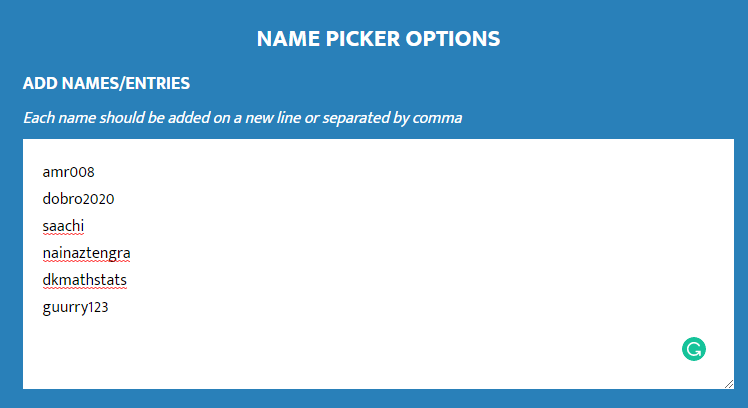And the Winner is::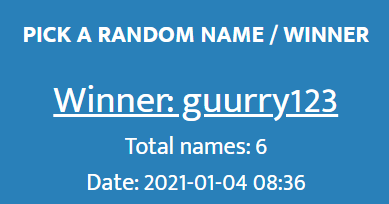Congratulations @guurry123; You WIN yourself 10 STEM tokens; worth 2.3+ HIVE. You should be having the rewards in your STEM Wallet Soon.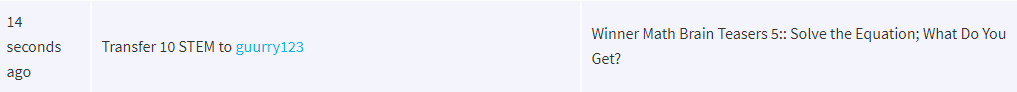Math Quote for the Day::

Here is the motivation to solve this Math Brain Teasers 6:: Solve the Picture Puzzle?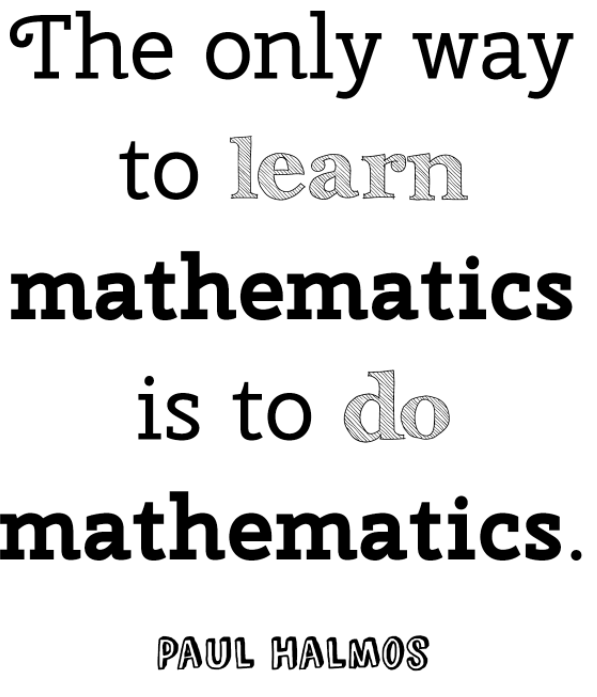source::

Best Regards

PS:: All the Maths Brain Teasers; are made by me using the Pro Canva License Version

Posted with STEMGeeks

Sort:

Not very sure but look like 15 to me.

Recalculating gives 13

last year (edited)

25
Thanks for the token

I guess it's 15 but the third image is confusing for me so not sure.

My answer is 25 for this. This one is slightly confusing.

13

is 13

Posted using Dapplr

last year (edited)

for me is 13

I got 13.

@dkmathstats how you reach 13 ?

From the 3 chocolates = 27, one chocolate piece is 3.

In the third line equation you have 2 chocolates pieces x 2 cupcakes = 12. You have 2 x 3 x (2 cupcakes) = 12. This would be 6 x 2 cupcakes = 12 which leads to 2 cupcakes being equal to 2. One cupcake would be 1.

In line one, the equation is donut x donut x cupcake = 16. The cupcake value is 1 which makes the donut equal to 4.

Use the known values to solve the final line with order of operations in mind.

!BEER you are right !!!!

last year Reveal Comment